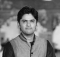## Counting substrings of a string that contains only one distinct letter in JavaScriptPublished on 20-Jan-2021 11:30:06
We are required to write a JavaScript function that takes in a string as the only argument. The task of our function is to count all the contiguous substrings in the input string that contains exactly one distinct letter.The function should then return the count of all such substrings.For example ... Read More

## Difference between product and sum of digits of a number in JavaScriptPublished on 20-Jan-2021 11:28:47
We are required to write a JavaScript function that takes in an positive integer as the only argument.The function should first count the sum of the digits of the number and then their product. Finally, the function should return the absolute difference of the product and the sum.For example −If ... Read More

## Pair of similar elements at different indices in JavaScriptPublished on 20-Jan-2021 11:27:01
We are required to write a JavaScript function that takes in an array of integers as the first and the only argument.The function is required to count the number of all such element pairs from the array that are equal in magnitude but are present at different indices.For example −If ... Read More

## Armstrong number within a range in JavaScriptPublished on 20-Jan-2021 11:25:19
Armstrong Numbers: A positive integer is called an Armstrong number (of order n) if −abcd... = a^n + b^n + c^n + d^n + ...We are required to write a JavaScript function that takes in an array of exactly two numbers specifying a range.The function should return an array of ... Read More

## Splitting array of numbers into two arrays with same average in JavaScriptPublished on 20-Jan-2021 11:23:23
We are required to write a JavaScript function that takes in an array of integers as the first and the only argument.The function needs to determine whether there exists a combination of elements of the input array that when they are divided into two groups (may/may not have equal elements), ... Read More

## Similar string groups (leetcode) in JavaScriptPublished on 20-Jan-2021 11:18:44
Two strings str1 and str2 are similar if we can swap two letters (in different positions) of str1, so that it equals str2. Also, two strings str1 and str2 are similar if they are equal.For example, "tars" and "rats" are similar (swapping at positions 0 and 2), and "rats" and ... Read More

## Finding missing element in an array of numbers in JavaScriptPublished on 20-Jan-2021 11:17:23
We are required to write a JavaScript function that takes in an array of numbers of length, say n. The array contains all the integers from 0 to n (including both 0 and n), but just one integer is missing, it can be any number and the array is not ... Read More

## Prime digits sum of a number in JavaScriptPublished on 20-Jan-2021 11:16:08
We are required to write a JavaScript function that takes in a number as the first and the only argument. The function should then sum all the digits of the number that are prime and return the sum as a number.For example −If the input number is −const num = ... Read More

## How to create permutation of array with the given number of elements in JavaScriptPublished on 20-Jan-2021 11:14:13
We are required to write a JavaScript function that takes in an array of literals as the first argument and a number as the second argument.The function should construct an array of all such arrays which have the length equal to the number specified by the second argument and contains ... Read More

## Adding numbers represented by string without complete conversion in JavaScriptPublished on 20-Jan-2021 11:12:43
We are required to write a JavaScript function that takes in two strings, str1 and str2 that represents two numbers.Without converting the whole strings to respective numbers, our function should calculate the sum of those two string numbers and return the result as a string.For example −If the two strings ... Read More